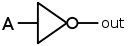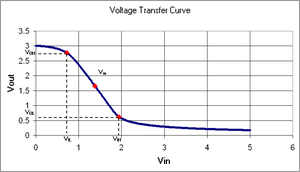# Inverter (logic gate)

Inverter (logic gate)
 INPUT A OUTPUT NOT A 0 1 1 0Traditional NOT Gate (Inverter) symbol

In digital logic, an inverter or NOT gate is a logic gate which implements logical negation. The truth table is shown on the right.

This represents perfect switching behavior, which is the defining assumption in Digital electronics. In practice, actual devices have electrical characteristics that must be carefully considered when designing inverters. In fact, the non-ideal transition region behavior of a CMOS inverter makes it useful in analog electronics as a class A amplifier (e.g., as the output stage of an operational amplifier).

## Electronic implementation

An inverter circuit outputs a voltage representing the opposite logic-level to its input. Inverters can be constructed using a single NMOS transistor or a single PMOS transistor coupled with a resistor. Since this 'resistive-drain' approach uses only a single type of transistor, it can be fabricated at low cost. However, because current flows through the resistor in one of the two states, the resistive-drain configuration is disadvantaged for power consumption and processing speed. Alternatively, inverters can be constructed using two complementary transistors in a CMOS configuration. This configuration greatly reduces power consumption since one of the transistors is always off in both logic states. Processing speed can also be improved due to the relatively low resistance compared to the NMOS-only or PMOS-only type devices. Inverters can also be constructed with Bipolar Junction Transistors (BJT) in either a resistor-transistor logic (RTL) or a transistor-transistor logic (TTL) configuration.

Digital electronics circuits operate at fixed voltage levels corresponding to a logical 0 or 1 (see Binary). An inverter circuit serves as the basic logic gate to swap between those two voltage levels. Implementation determines the actual voltage, but common levels include (0, +5V) for TTL circuits.

### Digital building blockThis schematic diagram shows the arrangement of NOT gates within a standard 4049 CMOS hex inverting buffer.

The digital inverter is considered the base building block for all digital electronics. Memory (1 bit register) is built as a latch by feeding the output of two serial inverters together. Multiplexers, decoders, state machines, and other sophisticated digital devices all rely on the basic inverter.

The Hex Inverter is an integrated circuit that contains six (hexa-) inverters. For example, the 7404 TTL chip which has 14 pins and the 4049 CMOS chip which has 16 pins, 2 of which are used for power/referencing, and 12 of which are used by the inputs and outputs of the six inverters (the 4049 has 2 pins with no connection).

### Performance measurement

Digital inverter quality is often measured using the Voltage Transfer Curve, which is a plot of input vs. output voltage. From such a graph, device parameters including noise tolerance, gain, and operating logic-levels can be obtained.Voltage Transfer Curve for a 20 μm Inverter constructed at North Carolina State University

Ideally, the voltage transfer curve (VTC) appears as an inverted step-function - this would indicate precise switching between on and off - but in real devices, a gradual transition region exists. The VTC indicates that for low input voltage, the circuit outputs high voltage; for high input, the output tapers off towards 0 volts. The slope of this transition region is a measure of quality - steep (close to -Infinity) slopes yield precise switching.

The tolerance to noise can be measured by comparing the minimum input to the maximum output for each region of operation (on / off).

The output voltage, VOH, can be a measure of signal driving strength when cascading many devices together.

Wikimedia Foundation. 2010.

### Look at other dictionaries:

• Logic gate — A logic gate is an idealized or physical device implementing a Boolean function, that is, it performs a logical operation on one or more logic inputs and produces a single logic output. Depending on the context, the term may refer to an ideal… …   Wikipedia

• Inverter — An inverter may refer to* Inverter, an electrical/electronic device which converts direct current (DC) to alternating current (AC) ** An Inverter tag found on some air conditioners indicates their ability to regulate the thermal power flow in a… …   Wikipedia

• Boolean algebra (logic) — For other uses, see Boolean algebra (disambiguation). Boolean algebra (or Boolean logic) is a logical calculus of truth values, developed by George Boole in the 1840s. It resembles the algebra of real numbers, but with the numeric operations of… …   Wikipedia

• And-inverter graph — An and inverter graph (AIG) is a directed, acyclic graph that represents a structural implementation of the logical functionality of a circuit or network. An AIG consists of two input nodes representing logical conjunction, terminal nodes labeled …   Wikipedia

• Four-phase logic — is a type of, and design methodology for, dynamic logic; it enabled non specialist engineers to design quite complex ICs, using either PMOS or NMOS processes.HistoryIn 1968 Lee Boysel [http://en.wikipedia.org/wiki/Four Phase Systems AL1] ,… …   Wikipedia

• Transistor–transistor logic — (TTL) is a class of digital circuits built from bipolar junction transistors (BJT), and resistors. It is called transistor–transistor logic because both the logic gating function (e.g., AND) and the amplifying function are performed by… …   Wikipedia

• Domino logic — is a CMOS based evolution of the dynamic logic techniques which were based on either PMOS or NMOS transistors. It allows a rail to rail logic swing. It was developed to speed up circuits. In Dynamic Logic, a problem arises when cascading one gate …   Wikipedia

• Integrated injection logic — (IIL, I2L, or I2L) is a class of digital circuits built with multiple collector bipolar junction transistors (BJT). When introduced it had speed comparable to TTL yet was almost as low power as CMOS, making it ideal for use in VLSI (and larger)… …   Wikipedia

• Pass transistor logic — In electronics, pass transistor logic (PTL) describes several logic families used in the design of integrated circuits. It reduces the count of transistors used to make different logic gates, by eliminating redundant transistors. Transistors are… …   Wikipedia

• Serie 4000 — La serie 4000, es una familia de la industria de circuitos integrados estándar que implementan una variedad de funciones lógicas. La también conocida como familia CMOS mejoras en algunas características a los circuitos integrados TTL de la serie… …   Wikipedia Español

### Share the article and excerpts

##### Direct link
Do a right-click on the link above
and select “Copy Link”